Wednesday 17th August 2022
 All India Engineering / Architecture Entrance Examination (AIEEE) CBSE Guess > AIEEE > AIEEE Papers > 2010 > Mathematics AIEEE 2010 Mathematics Q. 1.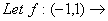R be a differentiable function with f(0)=-1and f’(0)=1,Let g(x)=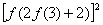.then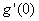–4 0 –2 4   Sol :Answer: (1) Q. 2. There are two urns. Urn A has 3 distinct red balls and urn B has 9 distinct blue balls. From each urn two balls are taken out at random and then transferred to the other. The number of ways in which this can be done is. 36 66 108 3   Sol : Total number of ways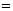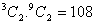Answer: (4)